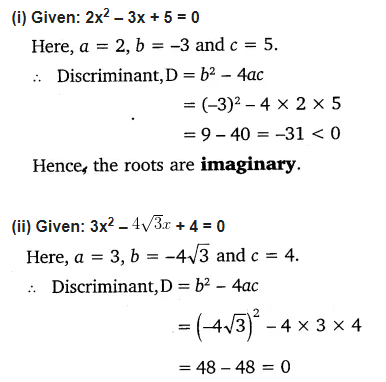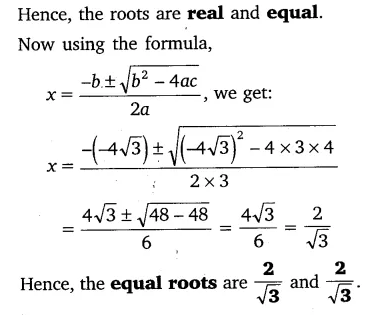# Find the nature of the roots of the following quadratic equations

Find the nature of the roots of the following quadratic equations. If the real roots exist, find them:
(i) 2x² -3x + 5 = 0
(ii) 3x2 –$4\sqrt { 3 } x$ + 4 = 0
(iii) 2x2-6x + 3 = 0